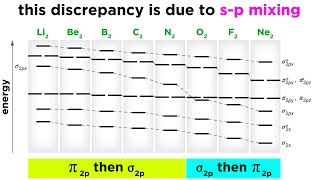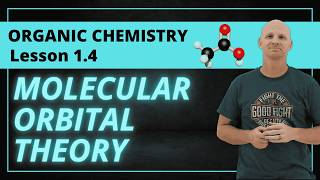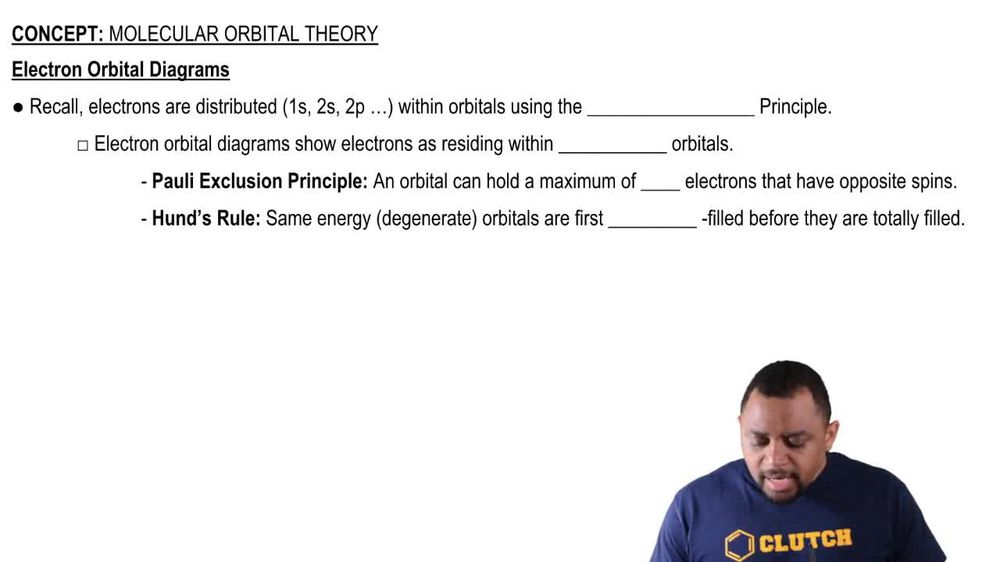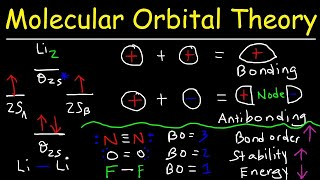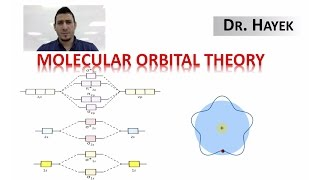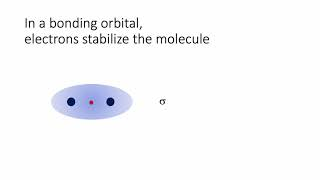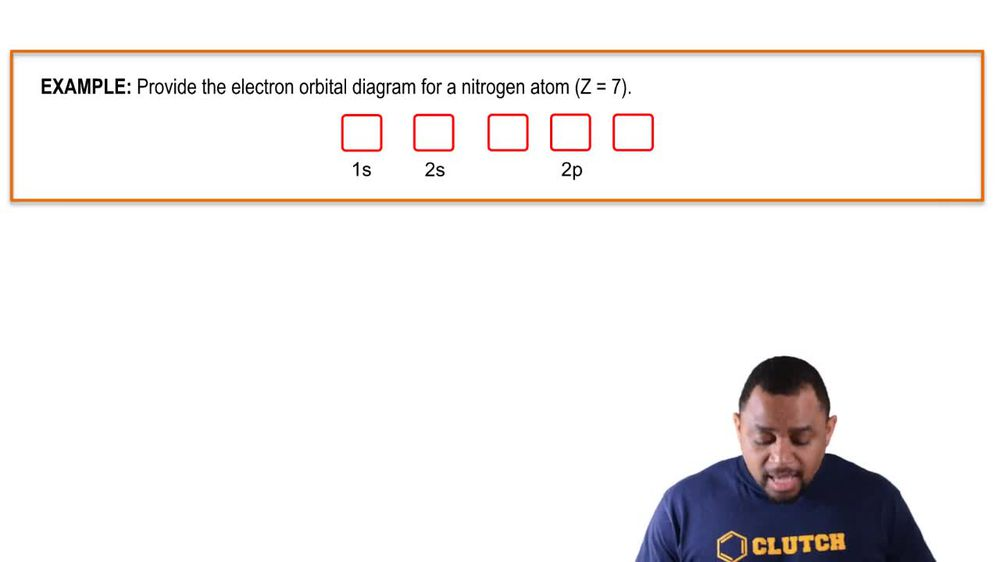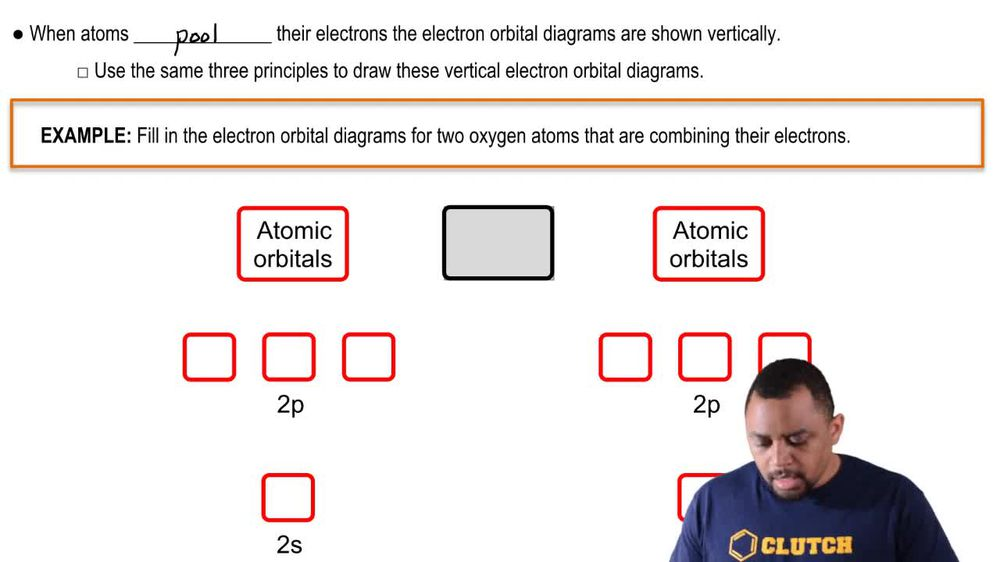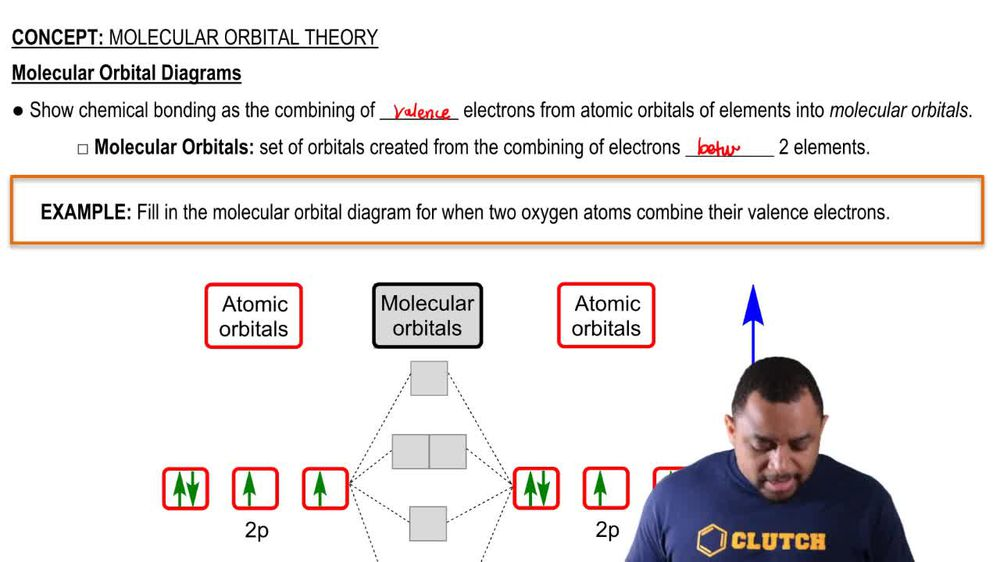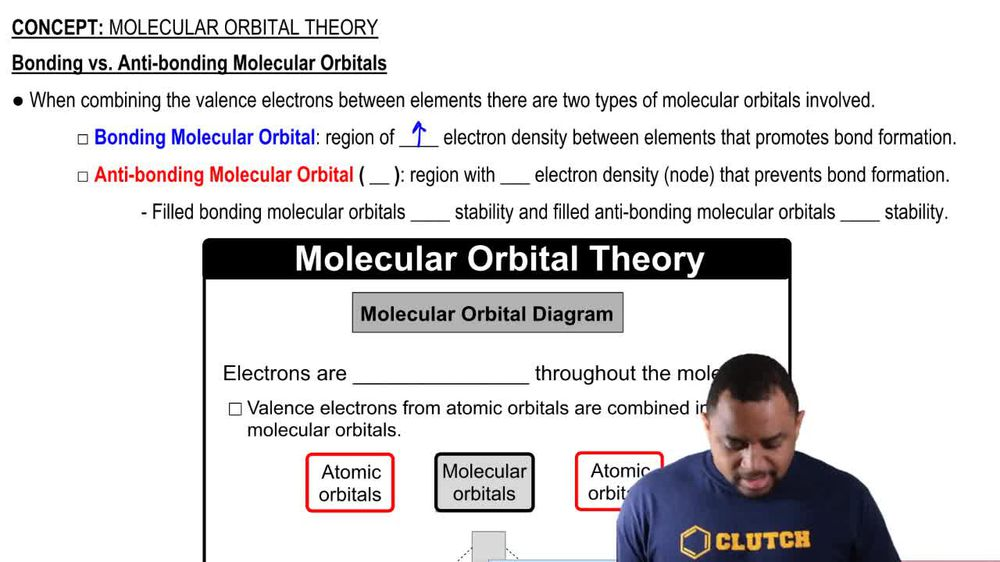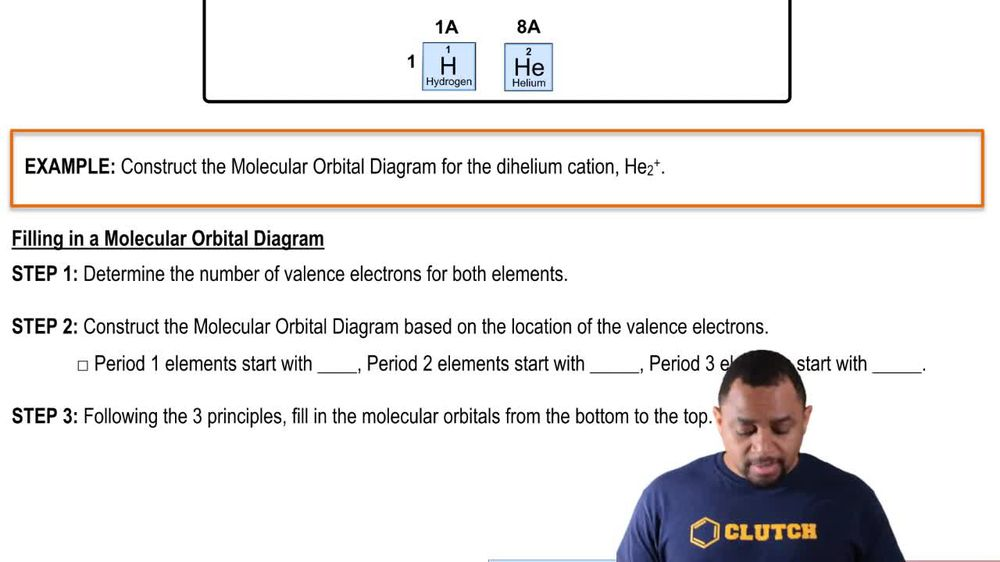Start typing, then use the up and down arrows to select an option from the list.
1. 12. Molecular Shapes & Valence Bond Theory2. Molecular Orbital Theory# Molecular Orbital Theory Example 4

by Jules Bruno
147 views
0
in this example. We need to construct the molecular orbital diagram for the dye helium cat ion, which is H E two plus. All right, so the way we do this is we're going to determine the number of valence electrons for both elements here. We're dealing with two helium. Remember he liam's have atomic numbers of two. We also say that helium, they each have two valence electrons. So they meet two times four on two times two Which is four valence electrons. But then here we're going to say this plus one charge means we've lost an electron. So in total we have three valence electrons for the dichotomy in castile. What we do next is we construct the molecular orbital diagram based on the location of the valence electrons. If we're dealing with period one elements like we are here then we start out with one s if it's period two elements it's to us and if it's period three it's three us Step three, we follow the three principles sold off Bob principle, Pauli exclusion principle and hunts rule and fill in the molecular orbital from bottom to the top. If we come back up here to our image here, we're dealing with three valence electrons. So we'd actually erase one of these and we have three total valence electrons which would then distribute into these molecular orbital's. So we started by filling out the lowest energy one according to off ball principle. So go one up one down and then we still have one more electron left that we need to fill in and we go here in the anti bonding molecular orbital. So this is what we would say are bonding molecular orbital diagram would resemble it will look like this. Okay. Where we would fill in totally be bonding molecular orbital that is sigma one S. And have half filled my anti bonding molecular orbital, which is sigma star one S.